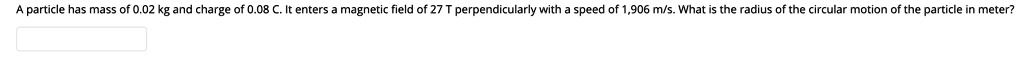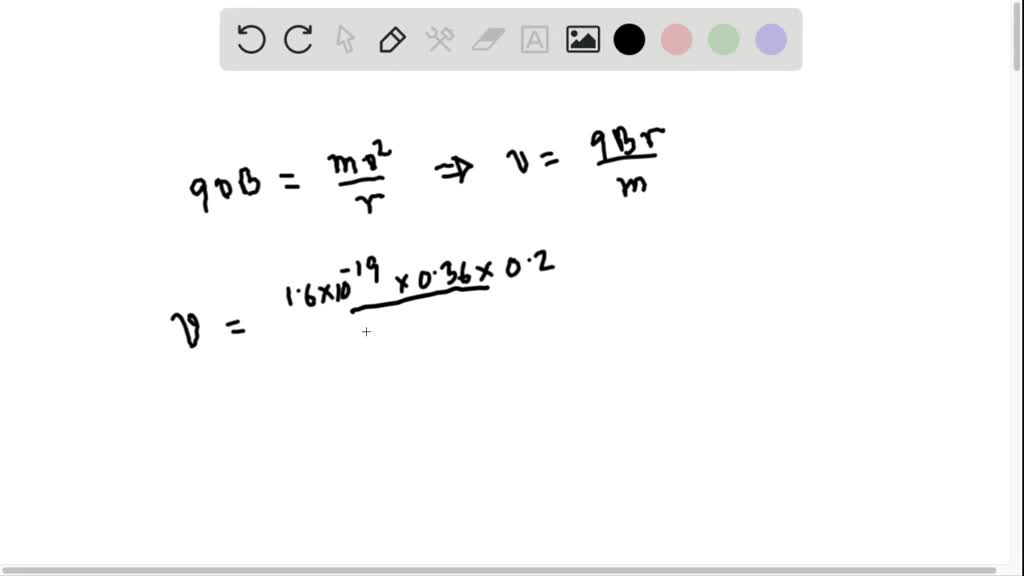5

# Particle has mass of 0.02 kg and charge of 0.08enters magnetic field of 27 perpendicularly with speed of 906 m/s. What is the radius of the circular motion of the p...

## Question

###### Particle has mass of 0.02 kg and charge of 0.08enters magnetic field of 27 perpendicularly with speed of 906 m/s. What is the radius of the circular motion of the particle in meter?

particle has mass of 0.02 kg and charge of 0.08 enters magnetic field of 27 perpendicularly with speed of 906 m/s. What is the radius of the circular motion of the particle in meter?